Powers of 10 Math Video for Kids - 3rd, 4th, & 5th Grades
1%
It was processed successfully!WHAT ARE POWERS OF 10?

A power of 10 is 10 multiplied by itself any number of times, such as 10 × 10 × 10. Powers of 10 can be written using exponential notation. 104 shows 10 being multiplied by itself 4 times.

To better understand powers of 10…

WHAT ARE POWERS OF 10?. A power of 10 is 10 multiplied by itself any number of times, such as 10 × 10 × 10. Powers of 10 can be written using exponential notation. 104 shows 10 being multiplied by itself 4 times. To better understand powers of 10…

## LET’S BREAK IT DOWN!

### Suitcase of Money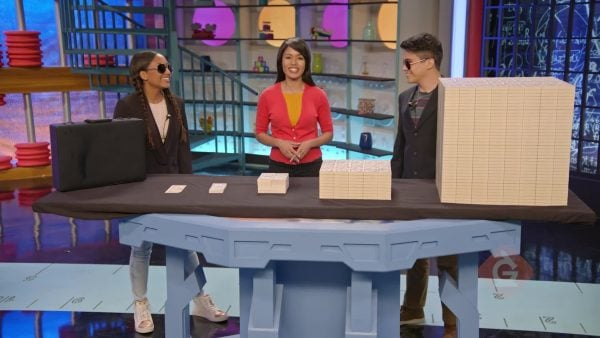Let's say we want to represent a million dollars using powers of 10. Start by making a pile with ten one-dollar bills, which represents \$10. Next, make another pile with ten times as many dollar bills or 10 × 10 dollars, which is \$100. The next pile has 10 × 10 × 10 dollar bills, or \$1,000. Each new pile has ten times as many dollar bills as the last pile, so our next pile has 10 × 10 × 10 × 10 dollar bills, or \$10,000, and the next pile has 10 × 10 × 10 × 10 × 10 bills, which is \$100,000. Finally, the last pile of dollar bills has 10 × 10 × 10 × 10 × 10 × 10, which is \$1,000,000. Each time we multiply by 10, the digits in our number shift one place value to the left. When working with large numbers, it is sometimes more convenient to represent these numbers in another form, called powers of 10. Our first pile of bills—which was \$10—can be represented as 10 to the power of 1, or 101. The next pile of \$100 can be represented as 10 to the power of 2, or 102, since 100 = 10 × 10, or two 10s multiplied together. \$1,000 can be represented as 10 to the power of 3 or 103. \$10,000 can be represented as 10 to the power of 4 or 104. \$100,000 can be represented as 10 to the power of 5 or 105. \$1,000,000 can be represented as 10 to the power of 6 or 106. We can write numbers in three different ways: in standard form (1,000), expanded form (10 × 10 × 10), and exponential form (103). Try this one yourself: What are the three different ways to express one hundred dollars?

Suitcase of Money Let's say we want to represent a million dollars using powers of 10. Start by making a pile with ten one-dollar bills, which represents \$10. Next, make another pile with ten times as many dollar bills or 10 × 10 dollars, which is \$100. The next pile has 10 × 10 × 10 dollar bills, or \$1,000. Each new pile has ten times as many dollar bills as the last pile, so our next pile has 10 × 10 × 10 × 10 dollar bills, or \$10,000, and the next pile has 10 × 10 × 10 × 10 × 10 bills, which is \$100,000. Finally, the last pile of dollar bills has 10 × 10 × 10 × 10 × 10 × 10, which is \$1,000,000. Each time we multiply by 10, the digits in our number shift one place value to the left. When working with large numbers, it is sometimes more convenient to represent these numbers in another form, called powers of 10. Our first pile of bills—which was \$10—can be represented as 10 to the power of 1, or 101. The next pile of \$100 can be represented as 10 to the power of 2, or 102, since 100 = 10 × 10, or two 10s multiplied together. \$1,000 can be represented as 10 to the power of 3 or 103. \$10,000 can be represented as 10 to the power of 4 or 104. \$100,000 can be represented as 10 to the power of 5 or 105. \$1,000,000 can be represented as 10 to the power of 6 or 106. We can write numbers in three different ways: in standard form (1,000), expanded form (10 × 10 × 10), and exponential form (103). Try this one yourself: What are the three different ways to express one hundred dollars?

### Model Airline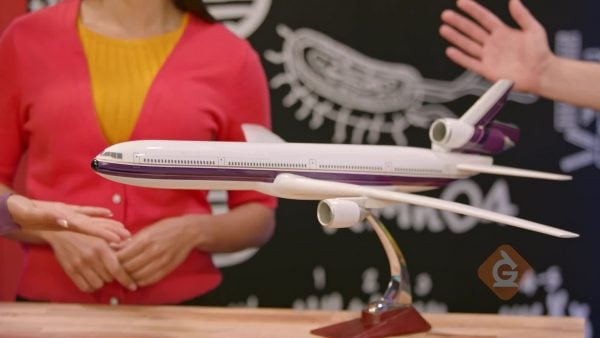Now let's explore how powers of 10 can help us multiply large numbers. Let's say that an airline has ten airplanes and each plane has 162 seats. How many seats is that in total? When you multiply a number by 10, also represented as 101, all of the digits in that number shift one place to the left. Following this pattern, the 1 is now in the thousands place, the 6 is in the hundreds place, and the 2 is in the tens place. Since we have no digit in the ones place, we put a zero there. This means that 10 times 162 is 1,620, and the airline has 1,620 total seats. Now let's say that each of those 1,620 passengers pays \$100 for their flight. How much money do the passengers pay in total? The number 100 can be represented as 10 to the power of 2, 102, or 10 × 10. When we multiply 1,620 by 100, we shift the digits two places to the left—the same number of places as the exponent (2), the number of times 10 is a factor in 10 × 10 (2), and the number of 0s in 100 (2). The 1 is now in the hundred thousands place, the 6 is in the ten thousands place, the 2 is in the thousands place, and the 0 is in the hundreds place, and since we have no tens or ones, we put zeros in both places. The total amount the passengers pay is \$162,000. Now, let's say that each flight uses 100,000 liters of fuel and the airline makes 21 flights a day. How much total fuel is that each day? To answer this, solve 21 × 100,000. It looks difficult, but 100,000 is the same as 10 to the power of 5, because it has 5 zeros. So, we shift the digits in the number 21 five places to the left. This gives us 2 in the millions place and 1 in the hundred thousands place, followed by 5 zeros. So the airline uses 2,100,000 liters of fuel each day. Try this yourself: If each of the 21 flights flies an average of 1,000 miles each day, how many total miles does the airline fly each day?

Model Airline Now let's explore how powers of 10 can help us multiply large numbers. Let's say that an airline has ten airplanes and each plane has 162 seats. How many seats is that in total? When you multiply a number by 10, also represented as 101, all of the digits in that number shift one place to the left. Following this pattern, the 1 is now in the thousands place, the 6 is in the hundreds place, and the 2 is in the tens place. Since we have no digit in the ones place, we put a zero there. This means that 10 times 162 is 1,620, and the airline has 1,620 total seats. Now let's say that each of those 1,620 passengers pays \$100 for their flight. How much money do the passengers pay in total? The number 100 can be represented as 10 to the power of 2, 102, or 10 × 10. When we multiply 1,620 by 100, we shift the digits two places to the left—the same number of places as the exponent (2), the number of times 10 is a factor in 10 × 10 (2), and the number of 0s in 100 (2). The 1 is now in the hundred thousands place, the 6 is in the ten thousands place, the 2 is in the thousands place, and the 0 is in the hundreds place, and since we have no tens or ones, we put zeros in both places. The total amount the passengers pay is \$162,000. Now, let's say that each flight uses 100,000 liters of fuel and the airline makes 21 flights a day. How much total fuel is that each day? To answer this, solve 21 × 100,000. It looks difficult, but 100,000 is the same as 10 to the power of 5, because it has 5 zeros. So, we shift the digits in the number 21 five places to the left. This gives us 2 in the millions place and 1 in the hundred thousands place, followed by 5 zeros. So the airline uses 2,100,000 liters of fuel each day. Try this yourself: If each of the 21 flights flies an average of 1,000 miles each day, how many total miles does the airline fly each day?

### Dog Washing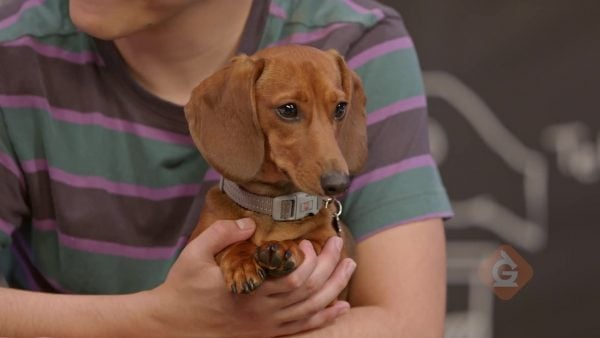Now, let's see how powers of 10 can help us multiply decimal numbers. Let's say you are washing dogs using a bucket that holds 6.85 liters of water. How many liters of water do 10 full buckets hold? Solve this problem by multiplying 10 × 6.85. When we multiply a number by ten, we shift each digit in that number one place to the left. The same pattern that we use to multiply a whole number by a power of ten works when we multiply a decimal number by a power of ten. Ten to the power of 1 times 6.85 equals 68.5, so ten buckets of water hold a total of 68.5 liters of water. Although it is the digits that move, another way to look at it is that the decimal shifts to the right one place. What if you use a bucket that holds 9.4 liters and fill it 100 times? How much water do you use? Solve the problem by multiplying 9.4 × 100. Since 100 is 10 to the power of 2, or 102, the digits in the number 9.4 shift two places to the left. The total is 940 liters of water. Try this yourself: How much water do we use if we fill a bucket that holds 12.5 liters of water 1,000 times?

Dog Washing Now, let's see how powers of 10 can help us multiply decimal numbers. Let's say you are washing dogs using a bucket that holds 6.85 liters of water. How many liters of water do 10 full buckets hold? Solve this problem by multiplying 10 × 6.85. When we multiply a number by ten, we shift each digit in that number one place to the left. The same pattern that we use to multiply a whole number by a power of ten works when we multiply a decimal number by a power of ten. Ten to the power of 1 times 6.85 equals 68.5, so ten buckets of water hold a total of 68.5 liters of water. Although it is the digits that move, another way to look at it is that the decimal shifts to the right one place. What if you use a bucket that holds 9.4 liters and fill it 100 times? How much water do you use? Solve the problem by multiplying 9.4 × 100. Since 100 is 10 to the power of 2, or 102, the digits in the number 9.4 shift two places to the left. The total is 940 liters of water. Try this yourself: How much water do we use if we fill a bucket that holds 12.5 liters of water 1,000 times?

### Sharing with Friends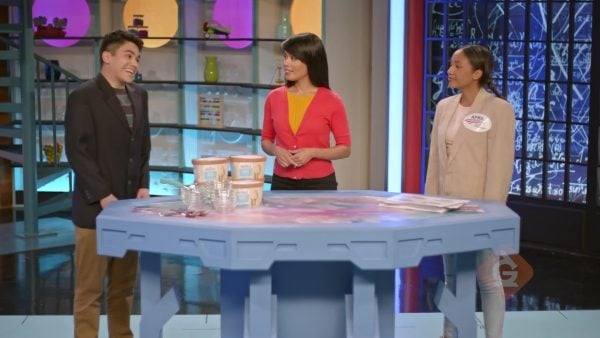Let's see how powers of 10 can help divide multi-digit whole and decimal numbers. If you have 800 stickers to divide among 10 friends, how many stickers does each friend receive? Solve this problem by dividing 800 by 10. When we divide by 10 to the power of 1, or 101, we are making the number 10 times smaller, or [ggfrac]1/10[/ggfrac] as big, so all of the digits shift one place to the right. That makes sense since division is the opposite of multiplication. To solve 800 divided by 10, move all of the digits one place to the right, so our answer is 80.0. Each of your ten friends gets 80 stickers. Now let's say you have 250 cups of ice cream to split equally between 100 students. How many cups of ice cream does each student get? To find out, solve 250 divided by 100. Since 100 is 10 to the power of 2, or 102, move the digits in the number 250 two places to the right. That means each student gets 2.5 cups of ice cream. Try this yourself: If you have 50 cups of ice cream to split equally between 100 students, how many cups does each student get?

Sharing with Friends Let's see how powers of 10 can help divide multi-digit whole and decimal numbers. If you have 800 stickers to divide among 10 friends, how many stickers does each friend receive? Solve this problem by dividing 800 by 10. When we divide by 10 to the power of 1, or 101, we are making the number 10 times smaller, or [ggfrac]1/10[/ggfrac] as big, so all of the digits shift one place to the right. That makes sense since division is the opposite of multiplication. To solve 800 divided by 10, move all of the digits one place to the right, so our answer is 80.0. Each of your ten friends gets 80 stickers. Now let's say you have 250 cups of ice cream to split equally between 100 students. How many cups of ice cream does each student get? To find out, solve 250 divided by 100. Since 100 is 10 to the power of 2, or 102, move the digits in the number 250 two places to the right. That means each student gets 2.5 cups of ice cream. Try this yourself: If you have 50 cups of ice cream to split equally between 100 students, how many cups does each student get?

## POWERS OF 10 VOCABULARY

Place value
The numerical value that a digit has based on its position in a number.
Exponent
A small raised number used in exponential notation to tell how many times the base is used as a factor. For example, in 103 the base is 10, the exponent is 3, and 103 = 10 x 10 x 10 = 1,000. Same as a power.
Power of 10
A way to write large numbers written using ten to a power, or exponent.
Base ten
A number system in which each number is 10 times the value to the right of it.
Digit
Any one of the symbols 0, 1, 2, 3, 4, 5, 6, 7, 8, and 9 in the base-ten number system.
Standard notation
A number written in the standard format, such as 100.
A number written as a word, such as one hundred.
A number written as repeated multiplication, such as 10 × 10.
A way to write repeated multiplication using a base and exponent. For example, in the exponential expression 102, the base 10 is used as a factor 2 times to represent 10 × 10 = 100.

## POWERS OF 10 DISCUSSION QUESTIONS

### When you multiply a number by a power of 10, do the digits in the number shift right or left? Explain.

The digits shift to the left because the number gets bigger.

### When you divide a number by a power of 10, do the digits in the number shift right or left? Explain.

The digits shift to the right because the number gets smaller.

### How does the exponent tell you how digits move when you multiply or divide by a power of 10?

The digits move the number of spaces equal to the whole number exponent.

104

### Explain what happens to the digits in the number 765.4 when you find the quotient 765.4 ÷ 10.

The digits all move one place to the right. [Alternatively, the decimal moves one place to the left.]
X

## Success

We’ve sent you an email with instructions how to reset your password.
Ok

## Choose Your Free Trial Period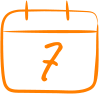### 7 DaysContinue to Lessons### 90 DaysGet 90 days free by inviting other teachers to try it too.

Share with Teachers

## Get 90 Days Free

### By inviting 4 other teachers to try it too.

4 required

Skip, I will use a 7 days free trial

## Thank You!

Enjoy your free 90 days trial